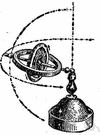# angular momentum

(redirected from Rotational momentum)
Also found in: Thesaurus, Medical, Encyclopedia.

## angular momentum

n.
1. The vector product of the position vector (from a reference point) and the linear momentum of a particle.
2. The vector sum of the angular momentums of each infinitesimal component particle of an extended body.

## angular momentum

n
(Mathematics) a property of a mass or system of masses turning about some fixed point; it is conserved in the absence of the action of external forces

## an′gular momen′tum

n.
the product of the moment of inertia of a body about an axis and its angular velocity with respect to the same axis.
[1870–75]

## an·gu·lar momentum

(ăng′gyə-lər)
A quantity used to measure the motion of a body that is moving in a circle. The angular momentum depends on the mass and velocity of the body, and on the radius of the circle that it is moving along. Because the velocity of a body moving in a circle is always changing (since velocity is speed and direction), its angular momentum is also always changing.
ThesaurusAntonymsRelated WordsSynonymsLegend:
 Noun 1angular momentum - the product of the momentum of a rotating body and its distance from the axis of rotation; "any rotating body has an angular momentum about its center of mass"; "angular momentum makes the world go round"momentum - the product of a body's mass and its velocity; "the momentum of the particles was deduced from meteoritic velocities"
Translations
moment hybnosti
kotni moment
ugaoni moment
References in periodicals archive ?
She and her father developed the small toy that allows for experimentation with the effects of angular and rotational momentum and newtonian physics.
ALMA observations showed the gas outflow rotates in the same direction as the gas disk that surrounds the young star, supporting the idea that the outflow contributes significantly to the dissipation of rotational momentum.
Likewise the concurrent (minute) perturbation of the planet's rotational momentum will damp down toward a stable state of spinning by energy transfer processes from the systemic potential to the surroundings and vice versa until the net dissipation finally vanishes at the free energy minimum state.

Site: Follow: Share:
Open / Close